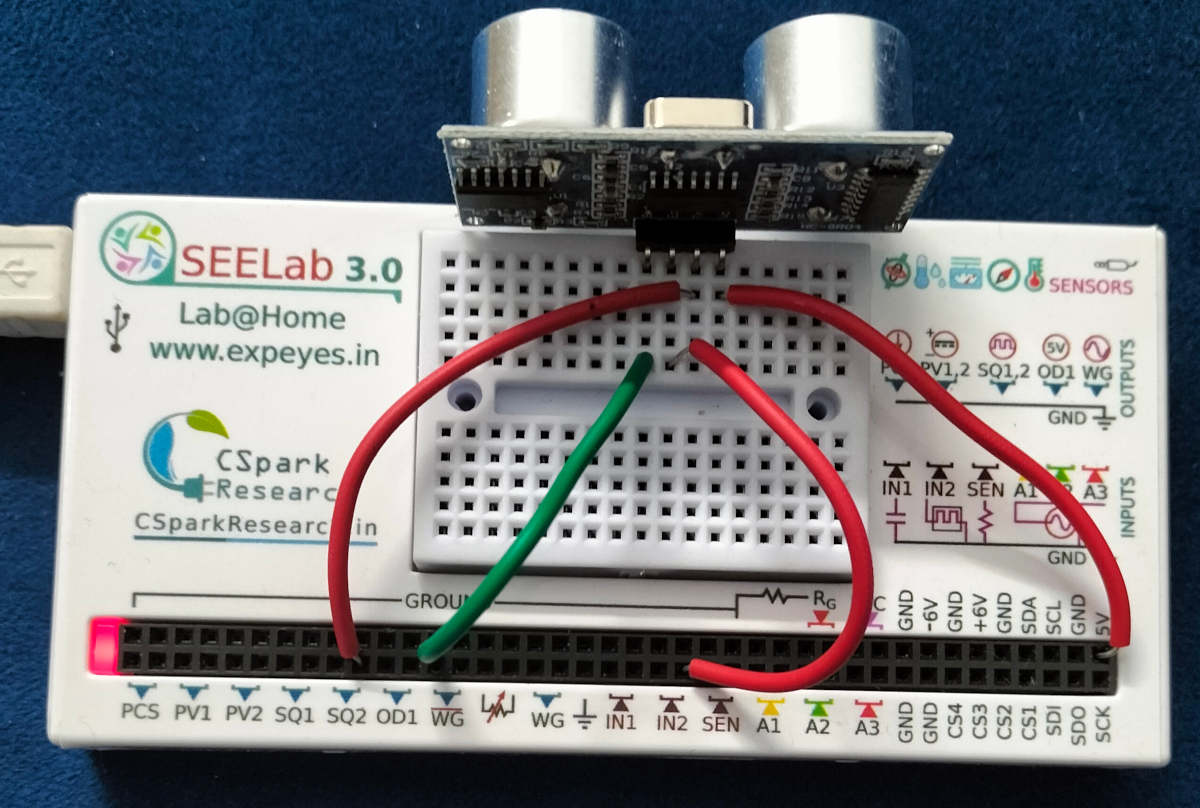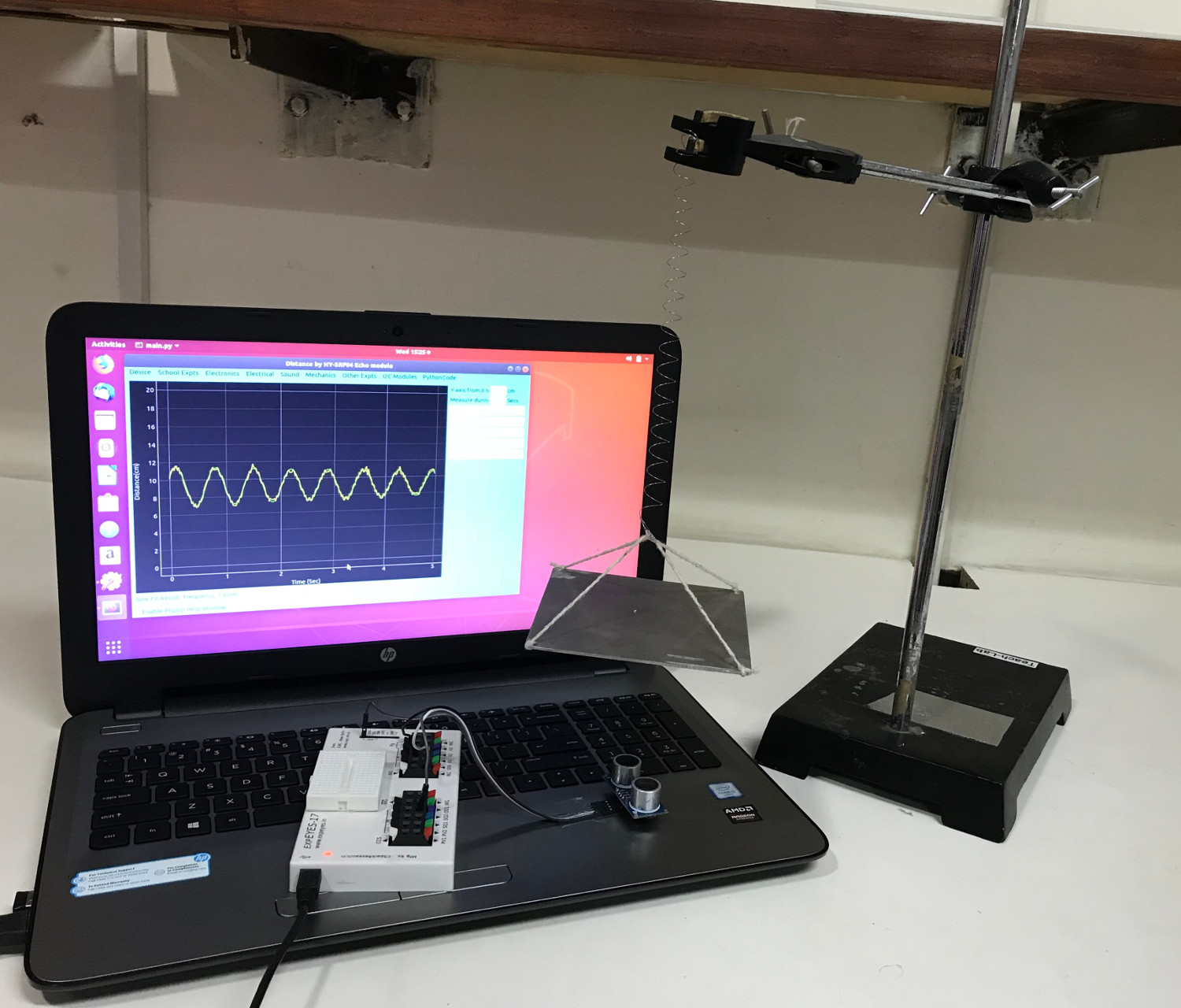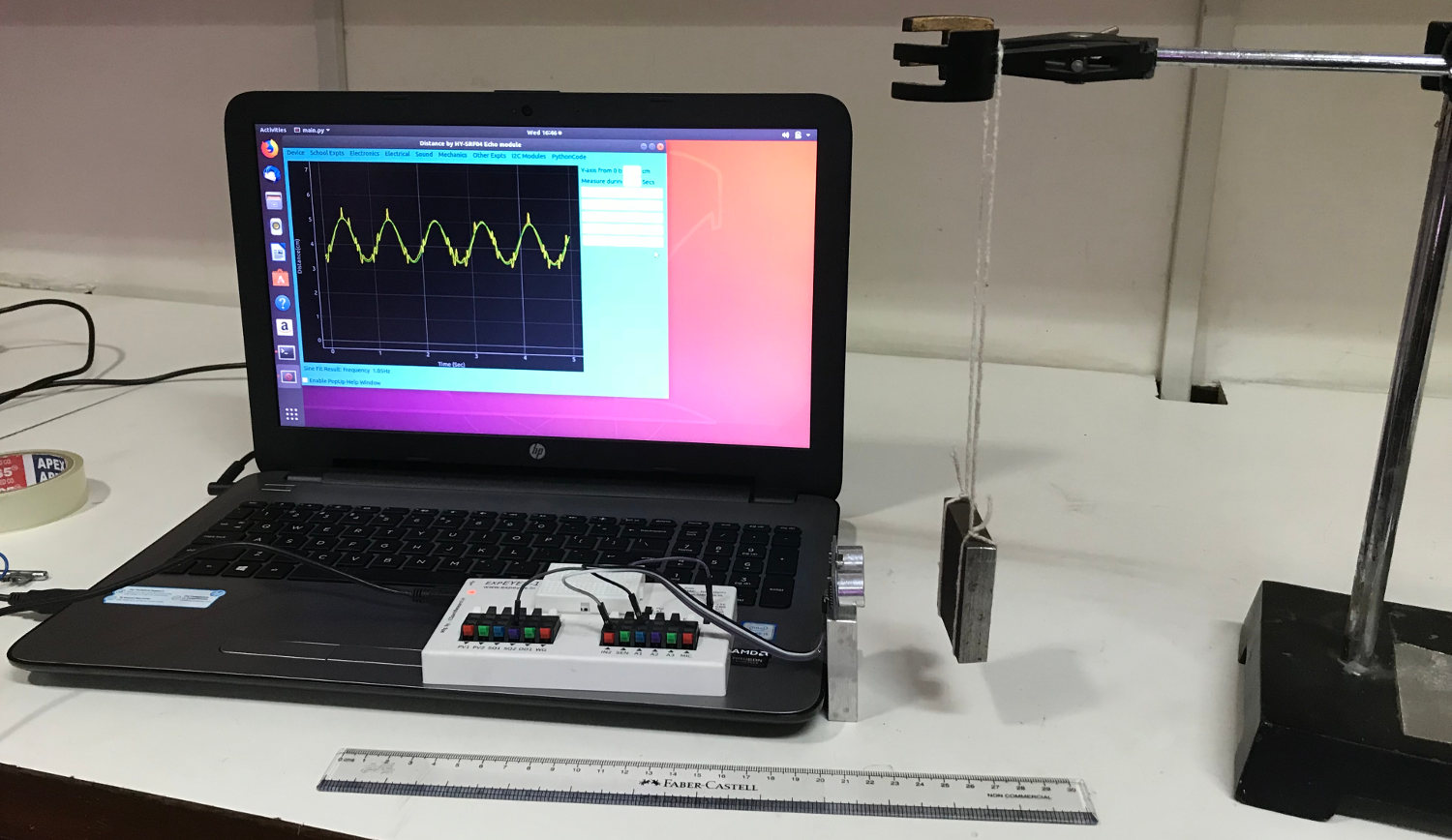# ExpEYES

## Mesuring distance using the SR04 ultrasound echo module

The connections are wired as shown in the figure below. An hard surface is placed in front of SR04 and moved back and forth. The distance from the sensor to the surface (that reflects the sound bursts from SR04) is recorded for 5 seconds. An area is selected and fitted using the sine function to get the frequency of oscillation. The results are displayed in the screenshot shown below.### The Mass and Spring systemWhen a mass suspended on a spring is made to oscillate, the period of oscillation is given by T = 2 * pi * sqrt( m/k), where m is the mass and k is the spring constant. We can measure the period of oscillation by measuring the distance to the moving mass as a function of time. The distance versus time graph is fitted with a sine function to calculate the frequency. The photograph below shows a metal plate suspended on a spring and the SR04 facing it from the bottom. The distance to the oscillating plate is measured for 5 seconds and the data is fitted to a sine function. A video of the experiment is HERE

### Pendulum

The period of oscillations of a pendulum also can be measured using SR04 connected to ExpEYES. The figure shows a pendulum with a rectangular bob, but one can use a spherical bob with a piece of paper (to provide a flat reflecting surface) pasted to it. The measured frequency is 1.05 Hz for a pendulum having a length of 22cm. One can calculate the value of acceleration due to gravity from this data.Watch the Video